# Totally ordered set

(diff) ← Older revision | Latest revision (diff) | Newer revision → (diff)
A partially ordered set in which for any two elementsandeitheror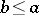. A subset of a totally ordered set is itself a totally ordered set. Every maximal (minimal) element of a totally ordered set is a largest (smallest) element. An important special case of totally ordered sets are the well-ordered sets (cf. Well-ordered set). Among the subsets of a partially ordered set that are totally ordered sets, a particularly important role is played by a composition sequence. A cut of a totally ordered setis a partition of it into two subsetsandsuch that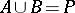,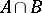is empty,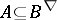and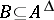, where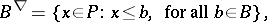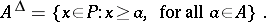The classesandare called the lower and upper classes of the cut. One can distinguish the following types of cuts: a jump — there is a largest element in the lower class and a smallest element in the upper class; a Dedekind cut — there is a largest (smallest) element in the lower (upper) class, but no smallest (largest) element in the upper (lower) class; a gap — there is no largest element in the lower class and no smallest element in the upper class. A totally ordered set is said to be continuous if all its cuts are Dedekind cuts. A subsetof a totally ordered setis said to be dense if every interval ofnot reducing to a single element contains elements belonging to. The totally ordered set of real numbers can be characterized as a continuous totally ordered set which has neither a largest nor a smallest element, but which contains a countable dense subset. Every countable totally ordered set is isomorphic to some subset of the totally ordered set of all binary fractions in the interval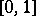. A latticeis isomorphic to a subset of the totally ordered set of integers if and only if every sublattice of it is a retract.